# Face Recognition Pipeline Clearly Explained

Introduction to State-of-the-art algorithm used in Face Recognition pipeline.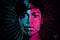image source

Face recognition is a technique of identification or verification of an individual using their face in a video or photo. This computer vision task captures, analyzes, and compares patterns based on the person’s facial details.

The 2011 book on face recognition titled “ Handbook of Face Recognition “ describes two main modes for face recognition, as:

A face recognition system is expected to identify faces present in images and videos automatically. …

# Kaggle Submission for Titanic Dataset

Exploratory Data Analysis and survival prediction with CatBoost algorithm.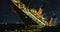Image from https://faithmag.com

Hello, data science enthusiast. In this blog post, I will guide through Kaggle’s submission on the Titanic dataset. We will do EDA on the titanic dataset using some commonly used tools and techniques in python. And then build some Machine Learning models to predict the target features. Want to revise what exactly EDA is? Here is my article on Introduction to EDA.

In one of my initial article Building Linear Regression Models, I explained how to model and predict different linear regression algorithm. In that case, the dataset I used had…

# Data Visualization with Python

visualization using python libraries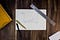Photo by Isaac Smith on Unsplash

Hello everyone! In this article, I will guide you through simple data visualization techniques in Python using different libraries like matplotlib, seaborn . I assume you already know about why data visualization is so important and why we do. So without doing any theoretical explanations, I would like to make you aware of a few facts.

Before visualizing data you should always identify which type of data you are working on? Is it a numerical variable or categorical? Even in numerical is it continuous or discrete type of data? First, you can select a few visualization…

# Introduction to Exploratory Data Analysis

Understand the concept of EDA in elementary words.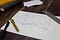Photo by Isaac Smith on Unsplash

While learning data science, Exploratory Data Analysis is the topic that you will encounter and also follow it but without exactly being aware of it (EDA). Exploratory Data Analysis, as its name suggest it is the technique of manipulating datasets, playing with it in right way, understand the datasets, represent the understandings, and modeling and implementing algorithms in order to understand the behavior of the data.

This definition seems like EDA and data mining/analysis are the same thing. …

# Building Linear Regression Models: modeling and predicting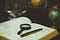Photo by João Silas on Unsplash

Hi everyone! In this post I will be giving you some insight on regression analysis and an experiment on a dataset that will make you aware about the concept of simple and multiple linear regression.

In this tutorial, we will be working with pandas, numpy, sklearn libraries and some visualization libraries like matplotlib and seaborn. Since you are into regression algorithms now you must have used these libraries for data analysis tasks before. If not, WHAT ARE PYTHON PACKAGES FOR DATA SCIENCE?## asha gaire

Practicing Data Science, AI Enthusiastic, Forthcoming ML Engineer